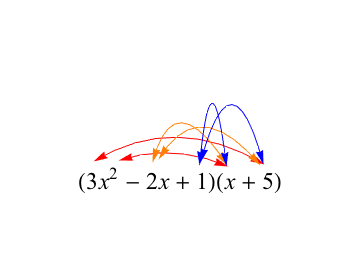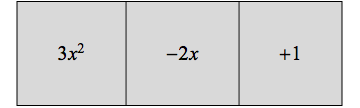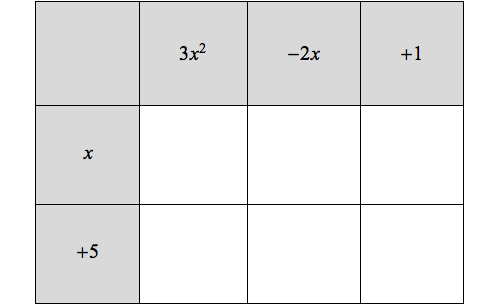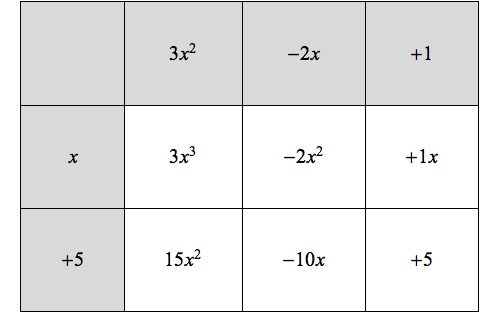Study Guide

# Performing Arithmetic Operations with Polynomials

## Performing Arithmetic Operations with Polynomials

High in the mountains on the planet of polynomials, we find our little friends hard at work arranging and rearranging themselves. Adding, subtracting, simplifying, and multiplying over and over again. Our friends (and we, too) must get in tip-top shape before our hike further up the mountain to search for factors and roots using the ever-elusive Remainder Theorem. Time for a workout.

• ### Adding and Subtracting Polynomials

Our polynomial calisthenics begin today with adding and subtracting. Like any exercise, we need to do it correctly for it to help. Thankfully, our polynomial friends promise to share their little tricks that make things much easier.The first trick is the ability to recognize and collect like terms. Like terms are little pieces of polynomials that have the same variables with the same exponents, but may differ in their numerical coefficients out front. For example, 2x and 3x are like terms, and 4ab2 and 2ab2 are like terms, too. Like terms are similar to paintings: they may look different up front, but they all look the same in the back.

To add or subtract like terms, we simply add or subtract the numerical coefficients. The trick here is we can only change the numerical coefficients out in front. Don't get carried away and start playing with the exponents.

The second little trick is our ability to remove parentheses. Remember our discoveries from our earlier visit to the planet of polynomials? If a set of parentheses has a "+" sign in front of it, the parentheses can simply be removed. Like this:

2x + (3x – 4y) =

2x + 3x – 4y

If the parentheses have a "–" sign in front, we need to distribute a -1 to every term inside the parentheses.

2x – (3x – 4y)

Okay, mathronaut. Remove the parentheses and distribute our -1.

2x – 3x + 4y

Time for a good workout with our little friends to build some muscles.

### Sample Problem

Add 2x – 6yz and 3x + 7y – 2z.

First, we rewrite it purely like a math problem. There are no words allowed between our numbers. We're being strict with our workout.

2x – 6yz + 3x + 7y – 2z

Now we look for like terms and group 'em together.

(2x + 3x) + (-6y + 7y) + (-z – 2z) =

5x + y – 3z

Excellent. We can feel the burn already.

### Sample Problem

Add 5m2n + 4mn – 8mn2 and 2m2nmnmn2.

Careful. We're excited, but we can't touch the exponents. They can be touchy at times. We can only add or subtract the numerical coefficients.

(5m2n + 2m2n) + (4mnmn) + (-8mn2mn2) =

7m2n + 3mn – 9mn2

Great. We're ready to ramp up the intensity to subtracting.

### Sample Problem

Subtract 4a2 – 7ab + b2 from 2a2ab – 6b2.

Put it all together.

2a2ab – 6b2 – (4a2 – 7ab + b2)

Remember that we're subtracting the entire first expression from the second, so we need to include those parentheses. Now, mathronauts, we remove the parentheses.

Easy, just like we learned in polynomial boot camp. Distribute that negative sign.

2a2ab – 6b2 – 4a2 + 7abb2

Now to collect like terms, and remember: no touching the exponents allowed. We're serious about that. The last time someone poked an exponent during our workout, it pushed over the exercise bike and started a fight with the polynomials. Never again.

(2a2 – 4a2) + (-ab + 7ab) + (-6b2b2) =

-2a2 + 6ab – 7b2

Good job, team. Now we and our little polynomials friends are ready for a challenge: simplifying.

### Sample Problem

Simplify 3x – [2y – (7zxy) – 4x + 3z].

We can do this. We just need to remember what we've learned about parentheses, and lifting.

Always start with the inner parentheses, and then we'll work our way to the outside ones.

3x – [2y – 7z + x + y – 4x + 3z

So far, so good. Now we need to remove the outer braces, switching everything's sign as we go because of the "–" sign out front.

3x – 2y + 7zxy + 4x – 3z

Not too shabby. We're almost done. We just need to collect the like terms.

(3xx + 4x) + (-2yy) + (7z – 3z) =

6x – 3y + 4z

Excellent. Man, look at us. We're totally ripped from all that polynomial exercise. It's now time to move on to the next set—multiplying.

• ### Multiplying Polynomials

Our polynomial friends are so excited. They have a few more tricks up their tiny little sleeves. Before we can head on up Polynomial Mountain, we need to use these tricks to master the art of multiplying.

The first little trick is called MNCAE: Multiply the Numerical Coefficients and Add Exponents (of like variables).

Or maybe it was MNCAE: My New Cat Ate Eggs. What does this have to do with the planet of polynomials? Absolutely nothing. We haven't even seen a cat here, but this will still help us remember how to multiply our polynomials.Time to try this out.

(2x3)(4x2)

Remember to MNCAE. Numbers are multiplied and exponents are added together. Multiply 2 × 4 = 8 and add 3 + 2 = 5.

8x5

One more time: MNCAE.

(3a2b4)(2ab5)

Multiply 3 × 2 = 6. Add 2 + 1 = 3 for the a terms, and add 4 + 5 = 9 for the b's.

6a3b9

We did it. Yes. It isn't enough, though. We want to pump *clap* you up.

### Sample Problem

Multiply a2 + a + 1 by a – 1.

We start by rewriting the problem as:

(a – 1)(a2 + a + 1)

We'll use the distributive property and MNCAE to lick this problem. Not literally, though. Gross.

a(a2 + a + 1) – 1(a2 + a + 1) =

a3 + a2 + aa2a – 1

Collect the like terms, and remember to only add and subtract the numerical coefficients.

a3 – 1

Another. We need another one. We're on a roll.

### Sample Problem

Simplify: (x + yz)(xy + z).

Go, My New Cat. Get that polynomial.

x(xy + z) + y(xy + z) – z(xy + z) =

x2xy + xz + xyy2 + yzxz + yzz2

As before, we collect the like terms, and don't touch the exponents. Never again, we said.

(x2) + (-xy + xy) + (xz xz) + (-y2) + (yz + yz) + (-z2) =

x2y2 + 2yz – z2

### Sample Problem

Multiply 3x2 + 2xy + y2 by x2 – 4xy + 2y2.

These expressions are getting more complicated, but there's still no worries.

(3x2 + 2xy + y2)(x2 – 4xy + 2y2)

We know the word that makes the problem go away. No, not the bird, MNCAE.

3x2(x2 – 4xy + 2y2) + 2xy(x2 – 4xy + 2y2) + y2(x2 – 4xy + 2y2) =

3x4 – 12x3y + 6x2y2 + 2x3y – 8x2y2 + 4xy3 + x2y2 – 4xy3 + 2y4

Okay, the problem doesn't go away immediately. In fact, it looks even bigger. Maybe we should have asked the bird instead. We can fix this, though. Just collect the like terms.

(3x4) + (-12x3y + 2x3y) + (6x2y2 – 8x2y2 + x2y2) + (4xy3 – 4xy3) + (2y4) =

3x4 – 10x3yx2y2 + 2y4

Whew. How about a couple more, before we start up Polynomial Mountain?

### Sample Problem

Multiply (5a – 2)(7a2 + 3a – 4).

We're so buff now from all this exercise. Maybe after we climb this mountain, we'll return to Earth and do a thousand push-ups to celebrate. That sounds like a good use of our newfound muscle.

5a(7a2 + 3a – 4) – 2(7a2 + 3a – 4) =

35a3 + 15a2 – 20a – 14a2 – 6a + 8

Oh yeah, the problem. Multiply coefficients, add exponents, collect like terms.

(35a3) + (15a2 – 14a2) + (-20a – 6a) + (8) =

35a3 + a2 – 26a + 8

We've got this down. It's time to hike on up the mountain.

### Sample Problem

Do you understand a concept better when there's a picture to help? We sure do. If so, multiplying polynomials will be the easiest procedure in all of math. Just use a table.

Think about this multiplication for a moment: (3x2 – 2x + 1)(x + 5). You could find the product using the rainbows of multiplication, which look something like this:Yeesh. It can be a little confusing. What a mess. After we draw our rainbows and multiply and double check that we didn't miss any terms, then we simplify.  Or we could not deal with any mess, and just make a picture and simplify. Let's try it.

First, make a table with as many columns as there are terms in your first polynomial. Write each term in its own column (in order, of course).Then, in the same table, make as many rows as there are terms in your second polynomial. In this case we get:And now, we just fill in the box like a multiplication table to find all our terms. Actually, it literally is a multiplication table.If we had forgotten to multiply two terms together, there would be an empty box. Remember: when we use this method, empty boxes are evil. Anyway, now that we have our terms, we write those terms in an expression and simplify.

3x3 – 2x2 + 1x + 15x2 – 10x + 5 =
3x3 + 13x2 – 9x + 5